# Acids and BasesPage 2

#### PREVIEW

Slide 10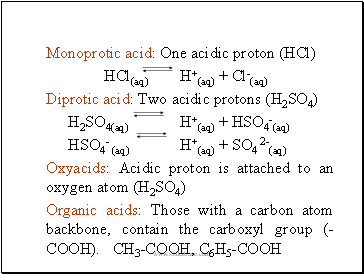Monoprotic acid: One acidic proton (HCl)

HCl(aq) H+(aq) + Cl-(aq)

Diprotic acid: Two acidic protons (H2SO4)

H2SO4(aq) H+(aq) + HSO4-(aq)

HSO4- (aq) H+(aq) + SO4 2-(aq)

Oxyacids: Acidic proton is attached to an oxygen atom (H2SO4)

Organic acids: Those with a carbon atom backbone, contain the carboxyl group (-COOH). CH3-COOH, C6H5-COOH

Slide 11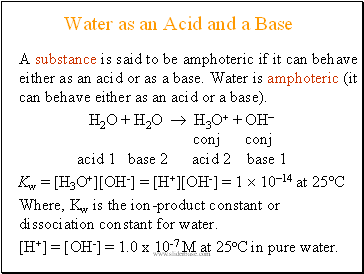## Water as an Acid and a Base

A substance is said to be amphoteric if it can behave either as an acid or as a base. Water is amphoteric (it can behave either as an acid or a base).

H2O + H2O  H3O+ + OH

conj conj

acid 1 base 2 acid 2 base 1

Kw = [H3O+][OH-] = [H+][OH-] = 1  1014 at 25°C

Where, Kw is the ion-product constant or dissociation constant for water.

[H+] = [OH-] = 1.0 x 10-7 M at 25oC in pure water.

Slide 12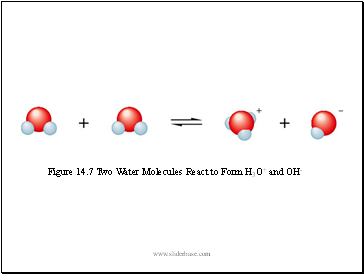Figure 14.7 Two Water Molecules React to Form H3O+ and OH-

Slide 13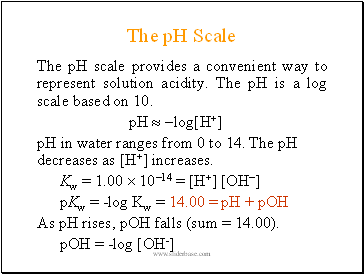## The pH Scale

The pH scale provides a convenient way to represent solution acidity. The pH is a log scale based on 10.

pH  log[H+]

pH in water ranges from 0 to 14. The pH decreases as [H+] increases.

Kw = 1.00  1014 = [H+] [OH]

pKw = -log Kw = 14.00 = pH + pOH

As pH rises, pOH falls (sum = 14.00).

pOH = -log [OH-]

Slide 14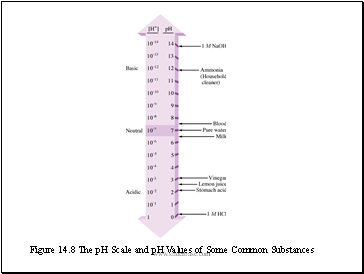Figure 14.8 The pH Scale and pH Values of Some Common Substances

Slide 15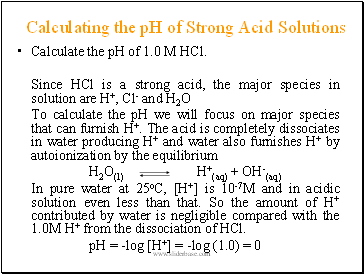## Calculating the pH of Strong Acid Solutions

Calculate the pH of 1.0 M HCl.

Since HCl is a strong acid, the major species in solution are H+, Cl- and H2O

To calculate the pH we will focus on major species that can furnish H+. The acid is completely dissociates in water producing H+ and water also furnishes H+ by autoionization by the equilibrium

H2O(l) H+(aq) + OH-(aq)

In pure water at 25oC, [H+] is 10-7M and in acidic solution even less than that. So the amount of H+ contributed by water is negligible compared with the 1.0M H+ from the dissociation of HCl.

pH = -log [H+] = -log (1.0) = 0

Slide 16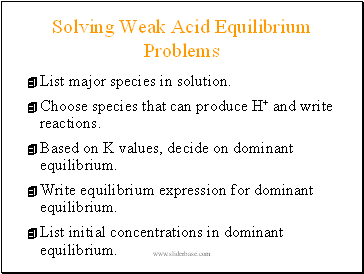## Solving Weak Acid Equilibrium Problems

List major species in solution.

Choose species that can produce H+ and write reactions.

Based on K values, decide on dominant equilibrium.

Write equilibrium expression for dominant equilibrium.

List initial concentrations in dominant equilibrium.

Go to page:
1  2  3  4